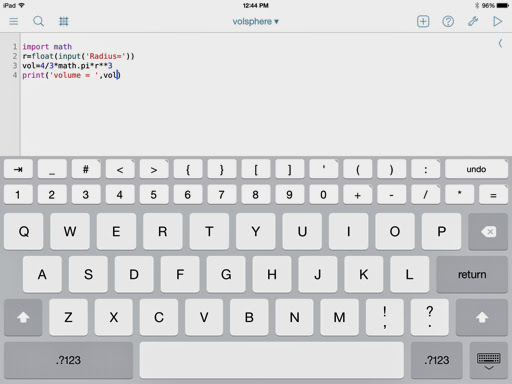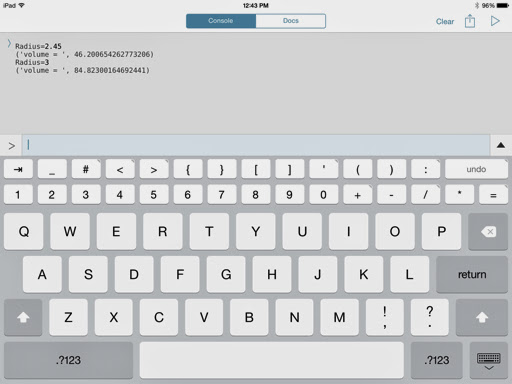## Tuesday, July 22, 2014

### My experience with Python so far (Pythonista iOS App 2.7)

Overall, my experience with Python has not been a great one, especially trying it with using iOS apps. (Update: but it's getting better - see below)

The iOS Python 3.2 app by Jonathan Hosmer crashes a lot on my iPad (3rd generation). Not worth the \$2.99 in my opinion.

I also tried the pythoni3.3 iOS app by XiaoWen Huang, which is free to download but has in app purchases. Sadly, this app fails in even in giving simple instructions as the help file will tell you everything of the history and how to use it on other machines, but forget it if you want to find how to invoke the interpreter on the app itself. And it crashes if I try to erase the word "in".

Then there is Pythonista 2.7 which has a great interface. However, I am not sure if the mathematics used by this app is not solid or I lack some obvious command. For instance:

Solving 2 by 2 Systems

Solve for x and y:
Ax + By = E
Cx + Dy = F

where:
x = (D*E-B*F)/(A*D-B*C)
y = (A*F-C*E)/(A*D-B*C)

Below is the code for it:
# solving 2 x 2 systems
print('Ax+By=E, Cx+Dy=F')
A=float(input('A='))
B=float(input('B='))
C=float(input('C='))
D=float(input('D='))
E=float(input('E='))
F=float(input('F='))
det=A*D-B*C
x=(D*E-B*F)/det
y=(A*F-C*E)/det
print(det)
print(x)
print(y)

Example:
A = 2
B = 3.5
C = -1
D = 0.4
E = 0.6
F = -2

The results I get are these:
x = 1.68372093023, y = -7.90697674429

Which is fine. I get comparable results when using other calculators and the MathStudio app to verify my answer.

However, the volume of a sphere tells another story.

Volume of the Sphere

Formula = 4/3 * π * r^3

Here is the program and results for r = 3. First the screenshots:Script:

import math
r=float(input('Radius='))
vol=4/3*math.pi*r**3
print('volume = ',vol)

r = 2.45; result Pythonista gives: 46.200654262773026

Verifying the answer with an fx-5800p and HP Prime I get: 61.60087235 (correct answer)

r = 3; result Pythonista gives: 84.82300164692441

However, my calculators return 113.0973355.

Again, am I missing something obvious? Or is Pythonista (maybe the language itself) not reliable?

(Updated) Thank you so much Bhuvanesh Bhatt!

Here is the corrected code:

import math
r=float(input('Radius='))
vol=4/3.*math.pi*r**3
print('volume = ',vol)

In version 2.7, which Pythonista runs, the slash operator (/) is treated as integer divsion. So a decimal point after the denominator is required in order to use floating divsion. In version 3, the extra decimal point is not required.

Under the updated code, I get the correct answers. Thanks once again Bhuvanesh!

Let me know your experiences in the comments below. Thanks as always, Eddie.

This blog is property of Edward Shore. 2014

#### 1 comment:

1.In Python 2.x, '/' does floor division for integers. For real division, change 4/3 to 4./3.

### Swiss Micros DM16L: Advanced Boolean and Factorial (up to 20)

Swiss Micros DM16L:   Advanced Boolean and Factorial (up to 20) Introduction The program listing, for the Swiss Micros DM16L and Hewlett Pac...